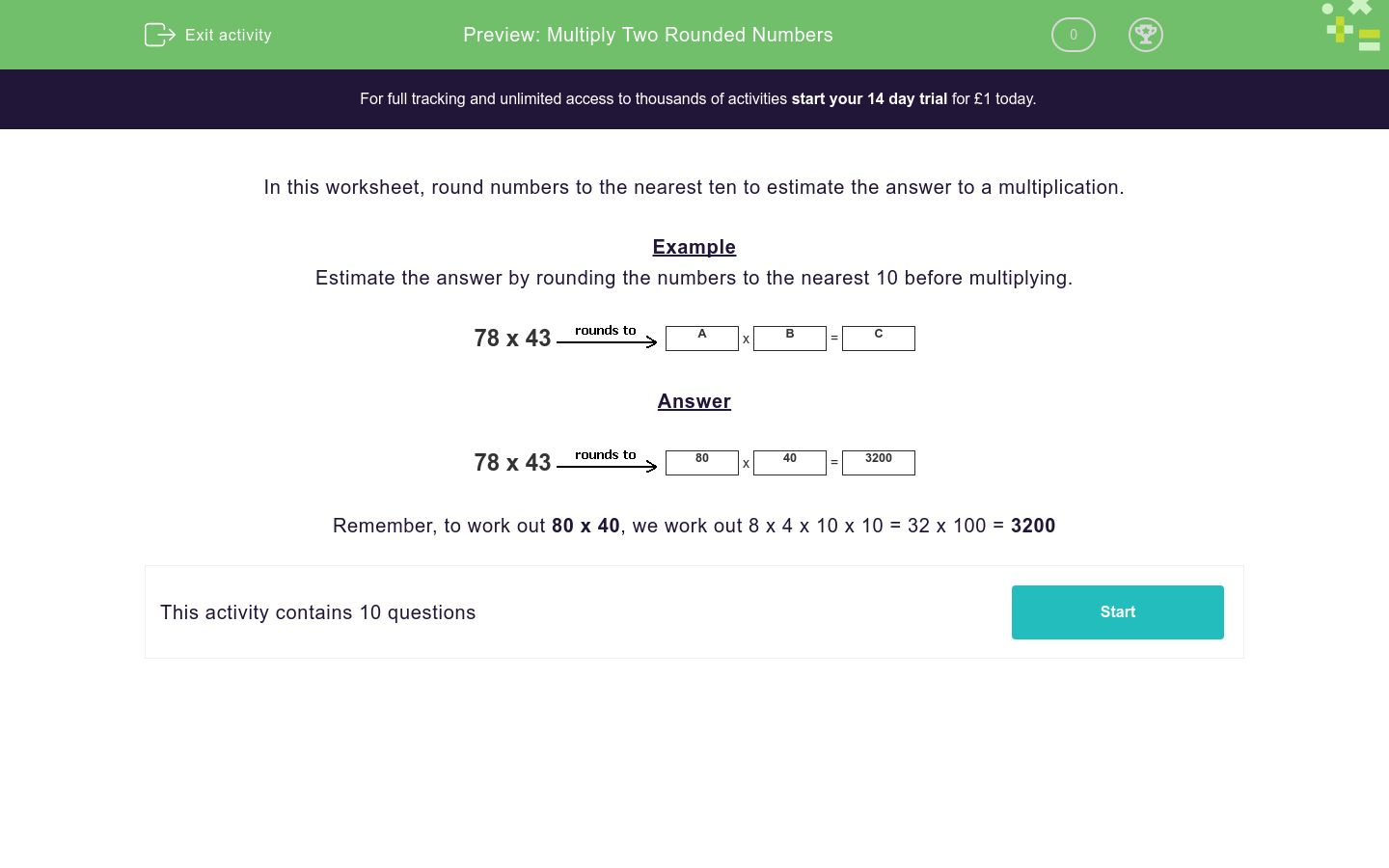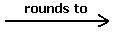# Multiply Two Rounded Numbers

In this worksheet, students use their rounding skills to round numbers to the nearest 10 before multiplying the numbers together.Key stage:  KS 2

Curriculum topic:   Number: Multiplication and Division

Curriculum subtopic:   Multiply Numbers to Four Digits

Difficulty level:### QUESTION 1 of 10

In this worksheet, round numbers to the nearest ten to estimate the answer to a multiplication.

Example

Estimate the answer by rounding the numbers to the nearest 10 before multiplying.

78 x 43A
x
 B
=
 C

78 x 4380
x
 40
=
 3200

Remember, to work out 80 x 40, we work out 8 x 4 x 10 x 10 = 32 x 100 = 3200

Estimate the answer by rounding the numbers to the nearest 10 before multiplying to find C.

67 x 32A
x
 B
=
 C

## Column B

A
70
B
30
C
2100

Estimate the answer by rounding the numbers to the nearest 10 before multiplying to find C.

87 x 43A
x
 B
=
 C

## Column B

A
3600
B
40
C
90

Estimate the answer by rounding the numbers to the nearest 10 before multiplying to find C.

22 x 32A
x
 B
=
 C

## Column B

A
30
B
20
C
600

Estimate the answer by rounding the numbers to the nearest 10 before multiplying to find C.

69 x 16A
x
 B
=
 C

## Column B

A
70
B
20
C
1400

Estimate the answer by rounding the numbers to the nearest 10 before multiplying to find C.

37 x 66A
x
 B
=
 C

## Column B

A
2800
B
40
C
70

Estimate the answer by rounding the numbers to the nearest 10 before multiplying to find C.

78 x 95A
x
 B
=
 C

## Column B

A
100
B
8000
C
80

Estimate the answer by rounding the numbers to the nearest 10 before multiplying to find C.

87 x 82A
x
 B
=
 C

## Column B

A
90
B
80
C
7200

Estimate the answer by rounding the numbers to the nearest 10 before multiplying to find C.

69 x 49A
x
 B
=
 C

## Column B

A
3500
B
50
C
70

Estimate the answer by rounding the numbers to the nearest 10 before multiplying to find C.

35 x 74A
x
 B
=
 C

## Column B

A
2800
B
40
C
70

Estimate the answer by rounding the numbers to the nearest 10 before multiplying to find C.

31 x 36A
x
 B
=
 C

## Column B

A
40
B
1200
C
30
• Question 1

Estimate the answer by rounding the numbers to the nearest 10 before multiplying to find C.

67 x 32A
x
 B
=
 C

## Column B

A
70
B
30
C
2100
EDDIE SAYS
70 x 30 = 2100
• Question 2

Estimate the answer by rounding the numbers to the nearest 10 before multiplying to find C.

87 x 43A
x
 B
=
 C

## Column B

A
90
B
40
C
3600
EDDIE SAYS
90 x 40 = 3600
• Question 3

Estimate the answer by rounding the numbers to the nearest 10 before multiplying to find C.

22 x 32A
x
 B
=
 C

## Column B

A
20
B
30
C
600
EDDIE SAYS
20 x 30 = 600
• Question 4

Estimate the answer by rounding the numbers to the nearest 10 before multiplying to find C.

69 x 16A
x
 B
=
 C

## Column B

A
70
B
20
C
1400
EDDIE SAYS
70 x 20 = 1400
• Question 5

Estimate the answer by rounding the numbers to the nearest 10 before multiplying to find C.

37 x 66A
x
 B
=
 C

## Column B

A
40
B
70
C
2800
EDDIE SAYS
40 x 70 = 2800
• Question 6

Estimate the answer by rounding the numbers to the nearest 10 before multiplying to find C.

78 x 95A
x
 B
=
 C

## Column B

A
80
B
100
C
8000
EDDIE SAYS
80 x 100 = 8000
• Question 7

Estimate the answer by rounding the numbers to the nearest 10 before multiplying to find C.

87 x 82A
x
 B
=
 C

## Column B

A
90
B
80
C
7200
EDDIE SAYS
90 x 80 = 7200
• Question 8

Estimate the answer by rounding the numbers to the nearest 10 before multiplying to find C.

69 x 49A
x
 B
=
 C

## Column B

A
70
B
50
C
3500
EDDIE SAYS
70 x 50 = 3500
• Question 9

Estimate the answer by rounding the numbers to the nearest 10 before multiplying to find C.

35 x 74A
x
 B
=
 C

## Column B

A
40
B
70
C
2800
EDDIE SAYS
40 x 70 = 2800
• Question 10

Estimate the answer by rounding the numbers to the nearest 10 before multiplying to find C.

31 x 36A
x
 B
=
 C

## Column B

A
30
B
40
C
1200
EDDIE SAYS
30 x 40 = 1200
---- OR ----

Sign up for a £1 trial so you can track and measure your child's progress on this activity.

### What is EdPlace?

We're your National Curriculum aligned online education content provider helping each child succeed in English, maths and science from year 1 to GCSE. With an EdPlace account you’ll be able to track and measure progress, helping each child achieve their best. We build confidence and attainment by personalising each child’s learning at a level that suits them.

Get started